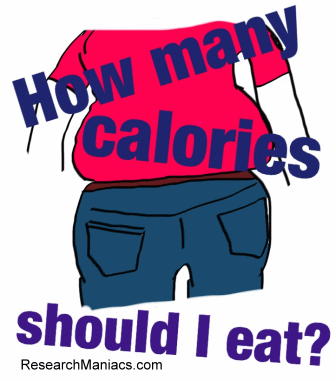How many calories should I eat?

Calculate how many calories you should eat?

How many calories should I eat, you may ask yourself. To find out how many calories you should eat, we need five pieces of information from you. We need your gender, height, weight, age, and how active you are.

What is your gender?   Female     Male

How tall are you?   Feet:     Inches:

How much do you weigh?   Pounds:

How old are you?  Years:

How active are you?

You should eat this many calories per day:

NOTE: This is how many calories you should eat per day if you are already the perfect weight for your body. Basically, you should eat the above amount of calories if you want to stay exactly the way you are now. If you eat more calories than the above on any given day, you will gain weight. If you eat less calories than the above number, then you will lose weight.

Losing weight is a simple matter of math!
3500 calories equals one pound. So, to lose a pound a week, you should eat 500 calories less per day than the number you calculated above. Simple as pie... or rather, simple as broccoli!

How did we calculate how many calories you should eat?

For females the formula is:
(655 + (4.35 x Pounds) + (4.7 x Height) - (4.7 x Age)) x Activity Level

For males, the formula is:
(66 + (6.23 x Pounds) + (12.7 x Height) - (6.8 x Age)) x Activity Level

The activity levels range from 1.2 to 1.9.

Of course, all bodies are different and your actual calorie need may be different than this simplified formula. The more active you are, the more you can eat. Also, do not try to lose more than 1 pound/week. You should not starve yourself! Your body will go into starvation mode, thinking food is scarce, and it will start to store fat, making it difficult to lose weight. Also remember that muscle burns calories 3 times faster than fat, so it's important to do muscle building exercises.

So, now you know how many calories you should eat. Good Luck!

Below are some other resources about weight, calories, and health that you may find useful.

 How much should I weigh? How much should I weighfor my height? How to Lose Weight This is how you lose weight for free. Research Maniacs' 4-step weight loss program. What is my BMI? Are you overweight? Calculate your BMI here! Why Eat Less Meat? You may want to cut down on all that red meat. Find out why here!Copyright  |   Privacy Policy  |   Disclaimer  |   Contact  |   Search